﻿ 基于CEEMDAN的时频峰值滤波微地震随机噪声压制方法
 石油地球物理勘探2021, Vol. 56Issue (2): 234-241  DOI: 10.13810/j.cnki.issn.1000-7210.2021.02.0030文章快速检索 高级检索

### 引用本文CHEN Yijun, CHENG Hao, GONG Enpu, XUE Lin. Suppression of random microseismic noise based on complete ensemble empirical mode decomposition with adaptive noise of TFPF. Oil Geophysical Prospecting, 2021, 56(2): 234-241. DOI: 10.13810/j.cnki.issn.1000-7210.2021.02.003.### 作者简介程浩, 辽宁省沈阳市和平区文化路三号巷11号东北大学资源与土木工程学院, 110819。Email: 872997349@qq.com

### 文章历史

Suppression of random microseismic noise based on complete ensemble empirical mode decomposition with adaptive noise of TFPF
CHEN Yijun , CHENG Hao, GONG Enpu , XUE Lin
Key Laboratory of Ministry of Education on Safe Mining of Deep Metal Mines, Northeastern University, Shenyang, Liaoning 110819, China
Abstract: Microseismic monitoring is widely applied in unconventional oil and gas fields, and supports the production and reserve increase of oil and gas fields. Because microseismic data are non-stationary, conventional denoising methods are not effective. This paper proposes a time-frequency peak filtering (TFPF) method of adaptive white noise based on the sample entropy (SE) complete set of empirical mode decomposition (CEEMDAN) to suppress noises while preserving effective signals. First raw microseismic data are decomposed into several IMFs of intrinsic modal components by CEEMDAN. Then after calculating the sample entropy, the IMFs are divided into two groups — one group will be filtered and the other will be left alone. The former group is TFPF filtered after selecting filter windows, and reconstructed with the latter to get final filtered signals. Application to theoretical model and field data has shown that the noise suppression method proposed in the paper is more effective than traditional EMD and constant-window TFPF denoising methods.
Keywords: microseism    denoising    time-frequency peak filtering (TFPF)    empirical mode decomposition (EMD)    sample entropy (SE)
0 引言

Huang等[9-11]的经验模态分解(EMD)方法是一种信号自适应分解方法，无需先验信息就能突显系统的物理特性。CEEMDAN(Complete ensemble EMD with adaptive noise)方法是对EMD方法的改进，利用白噪声均匀分布的统计特性，通过对目标信号多次添加一定幅值的白噪声来克服模态混叠的影响。但基于EMD的阈值去噪方法对于低信噪比地震记录滤波效果不佳。即使在信噪比为-9dB情况下，时频峰值滤波(TFPF)算法也可有效压制噪声。这两种方法现广泛应用于机器故障监测、信号处理、地震探测等领域。

1 基本原理 1.1 自适应白噪声完整集合经验模态分解

CEEMDAN通过在各阶段添加有限次的自适应白噪声，实现在较小平均次数下重构误差趋近于零。该算法在程序上实现了添加自适应白噪声，可很好地抑制模态混淆且避免原始信号失真。具体步骤如下。

(1) 在原始数据S(t)中分别多次添加自适应白噪声Bq(t)，其中q表示添加噪声次数，一般取10~50，本文取q=50，则第q次信号可表示为 $\boldsymbol{S}_{q}(t)=$$\boldsymbol{S}(t)+\alpha_{q} \boldsymbol{B}_{q}(t)(q=1,2, \cdots, 50) ，其中αq为第q次加入白噪声的标准差。CEEMDAN的一阶IMF分量为  {\rm{IM}}{{\rm{F}}_1} = \frac{1}{{50}}\sum\limits_{q = 1}^{50} {{\rm{IMF}}_1^{(q)}} (1) 式中IMF1(q)表示Sq(t)分解的一阶IMF。余项 \boldsymbol{R}_{1}(t)$$ =\boldsymbol{S}(t)-\mathrm{IMF}_{1}$

(2) 构造新的待分解信号 $\boldsymbol{S}(t), \boldsymbol{S}(t)=\boldsymbol{R}_{1}(t)+$$\alpha_{q} \boldsymbol{B}_{q}(t) ，进行到第50次分解，得到CEEMDAN的二阶IMF分量  {\rm{IM}}{{\rm{F}}_2} = \frac{1}{{50}}\sum\limits_{q = 1}^{50} {{\rm{IMF}}_2^{(q)}} (2) 余项 \boldsymbol{R}_{2}(t)=\boldsymbol{S}(t)-\mathrm{IMF}_{2} (3) 重复步骤(1)和步骤(2)，到程序终止，共产生w个IMF，最后的余项为  \boldsymbol{R}=\boldsymbol{S}(t)-\sum\limits_{p=1}^{w} \mathrm{IMF}_{p} (3) 1.2 样本熵分析 样本熵是一种与近似熵类似但精度更高的数据复杂度衡量指标，可对数据的复杂度进行量化分析。样本熵的物理意义是表征信号中产生新模式的概率大小，以衡量时间序列复杂性。即新模式产生概率越小——样本熵越小，序列自我相似度就越高；样本熵越大，样本序列就越复杂。本文中样本熵越大，IMFs中各个频率越多，具体体现为噪声含量高；样本熵越小，IMFs越趋向于有效信号。 通常，N个数据点组成的时间序列 \{\boldsymbol{x}(n)=x(1),x(2), \cdots, x(n)\} 的样本熵的计算包括如下步骤： (1) 按序号组成1组m维的向量序列： \boldsymbol{X}_{m}(1)$$\boldsymbol{X}_{m}(2), \cdots, \boldsymbol{X}_{m}(i), \cdots, \boldsymbol{X}_{m}(N-m+1)$, 其中 $\boldsymbol{X}_{m}(i)=$ $\{x(i), x(i+1), \cdots, x(i+m-1)\}, 1 \leqslant i \leqslant N-m+1$,，这些向量代表从第i个点开始的m个连续的值；

(2) 将向量 $\boldsymbol{X}_{m}(i)$ 与 $\boldsymbol{X}_{m}(j)$ 之间的距离定义为对应元素中最大差值的绝对值

 $d\left[\boldsymbol{X}_{m}(i), \boldsymbol{X}_{m}(j)\right]=\max [|x(i+k)-x(j+k)|]$ (4)

(3) 设相似容限(Tolerance for accepting matches)为r，通常取值为0.10~0.25个时间序列标准差。向量 $\boldsymbol{X}_{m}(i)$$\boldsymbol{X}_{m}(j)$ 的距离小于或等于r的数目为 $\boldsymbol{B}_{i}, i \neq j$ 。其均值定义为

 $\boldsymbol{B}_{i}^{m}(r)=\frac{\boldsymbol{B}_{i}}{N-m-1} \quad 1 \leqslant i \leqslant N-m$ (5)

(4) 对所有 $\boldsymbol{B}_{i}^{m}(r)$ 求均值，可得

 $\boldsymbol{B}^{m}(r)=\frac{1}{N-m} \sum\limits_{i=1}^{N-m} \boldsymbol{B}_{i}^{m}(r)$ (6)

(5) 将维数m增至m+1，重复以上步骤，可得$\boldsymbol{B}^{m+1}(r)$

(6) 当N为有限值时，定义样本熵

 $\operatorname{SampEn}(m, r, N)=-\ln \frac{\boldsymbol{B}^{m+1}(r)}{\boldsymbol{B}^{m}(r)}$ (7)
1.3 时频峰值滤波(TFPF) 1.3.1 基本原理

TFPF的本质是基于Wigner-Ville分布(WVD)的瞬时频率估计。待处理的含噪数据可表示为

 $\boldsymbol{s}(t)=\boldsymbol{x}(t)+\boldsymbol{n}(t)$ (8)

(1)对含噪数据进行编码，将其变为解析信号的形式；对含噪数据s(t)进行频率调制，得到单位幅度的解析信号

 $\boldsymbol{z}_{s}(t)=\exp \left[\mathrm{j} 2 \pi \mu \int_{0}^{t} \boldsymbol s(\lambda) \mathrm{d} \lambda\right]$ (9)

(2) 取解析信号z(t)的伪Wigner-Ville分布(PWVD)的峰值，对解析信号进行瞬时频率估计，作为有效信号x(t)的估计值

 $\hat{x}=\hat{f}(t)=\frac{\arg \max \left[W_{z}(t, f)\right]}{\mu}$ (10)

1.3.2 窗长选择对TFPF滤波的影响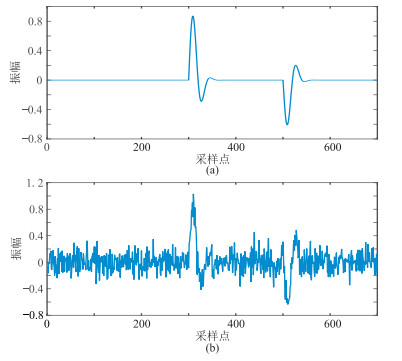图 1 有效信号(a)及其含噪数据(b)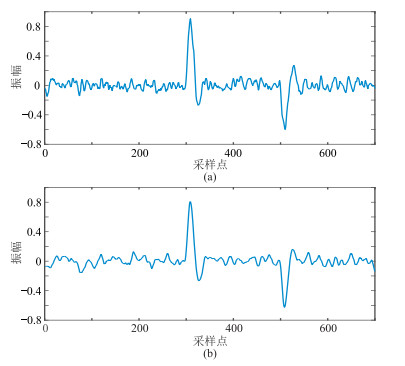图 2 窗长分别为9(a)和13(b)的滤波结果
2 基于CEEMDAN的TFPF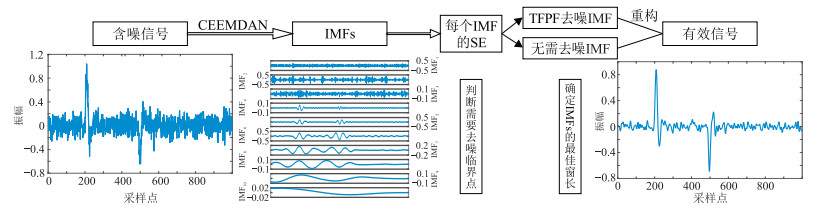图 3 本文方法处理流程图

(1) 利用CEEMDAN分解信号，能使信号按从高到低频率分解，得到若干个IMF分量。

(2) 通过计算IMFs的样本熵判断含噪临界点，高于此临界值的IMFs需进行滤波。

(3) 在选定需进行TFPF的IMFs后，根据地震数据的经验公式对各个IMF选择合适窗长

 $W_{L} \leqslant \frac{0.384 f_{\mathrm{s}}}{f_{\mathrm{d}}}$ (11)

(4) 最后将滤波后的IMFs与保留的IMFs重构，即可得到有效信号。

3 数值实验结果与分析 3.1 理论模型试算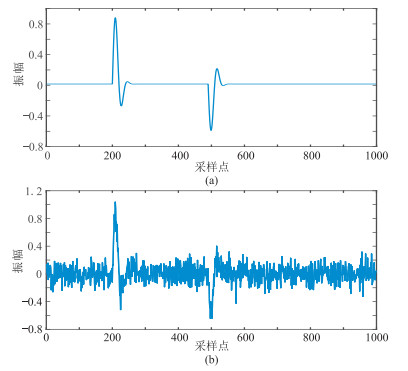图 4 理论数据模型 (a)原始数据；(b)加噪信号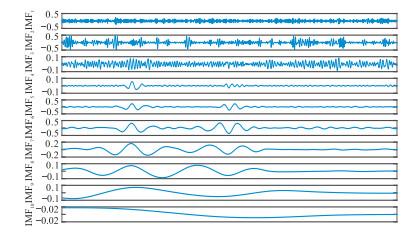图 5 理论模型数据经CEEMDAN分解后的IMFs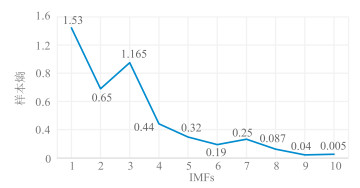图 6 图 5各个IMFs对应的样本熵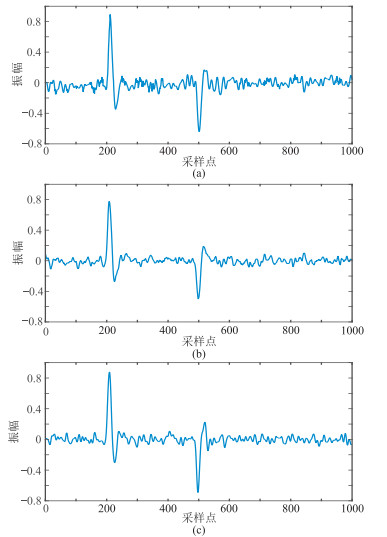图 7 理论模型数据不同方法去噪结果 (a)传统EMD；(b)固定窗长TFPF；(c)CEEMDAN-TFPF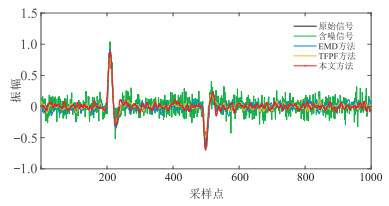图 8 不同方法去噪结果波形对比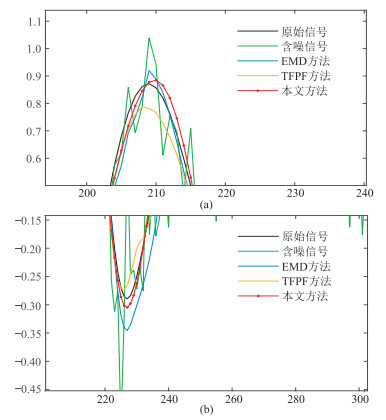图 9 对应图 8波峰(a)、波谷(b)波形的放大显示
3.2 实际微地震数据应用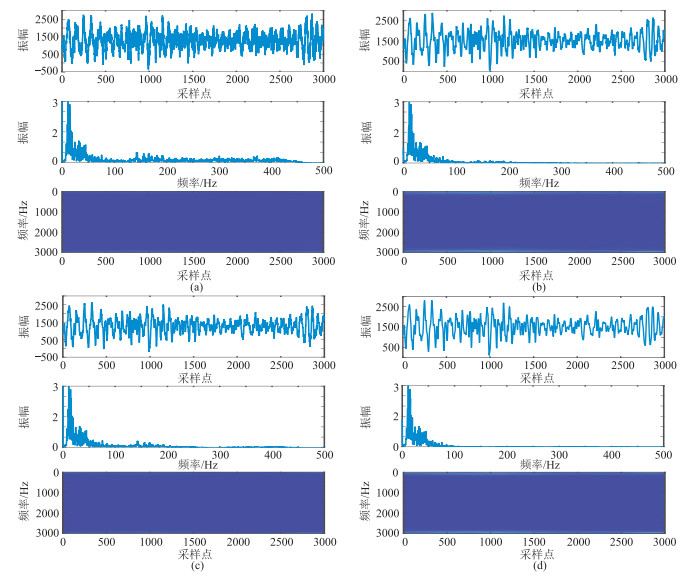图 10 针对实际微地震数据应用不同方法去噪所得波形、频谱及时频对比 (a)实际原始微地震数据；(b)传统EMD方法；(c)固定窗长TFPF方法；(d)本文(CEEMDAN-TFPF)方法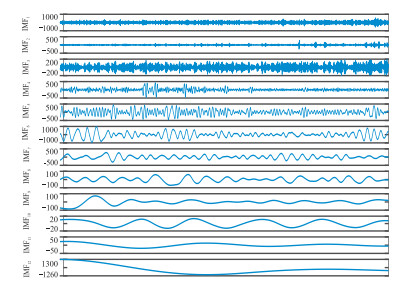图 11 实际微地震数据经CEEMDAN分解后的IMFs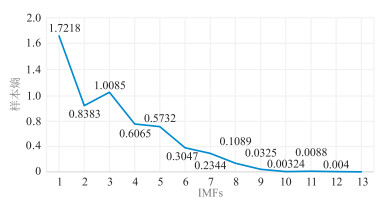图 12 实际微地震数据各IMF对应的样本熵

4 结论

(1) CEEMDAN-TFPF去噪方法是自适应的，对参数的选择无严格限制，能提高数据分析的效率。

(2) 该方法能有效克服EMD的模态混叠问题，且可更精准地判别噪声分量，对需滤波的IMF灵活选择窗长，达到信号保幅与噪声压制间的平衡，提高微地震数据信噪比。

(3) 与传统EMD和定窗长TFPF两种去噪方法相比，本文方法能在有效压制随机噪声的同时，也很好地保护有效信号幅值，准确识别有效微地震信号，提高定位精度，保留原始微地震事件的数据特征，给后续微地震数据研究提供可靠基础数据。

  邹才能, 杨智, 朱如凯, 等. 中国非常规油气勘探开发与理论技术进展[J]. 地质学报, 2015, 89(6): 979-1007. ZOU Caineng, YANG Zhi, ZHU Rukai, et al. Progress in China's unconventional oil & gas exploration and development and theoretical technologies[J]. Acta Geologica Sinica, 2015, 89(6): 979-1007. DOI:10.3969/j.issn.0001-5717.2015.06.001  董世泰, 高红霞. 微地震监测技术及其在油田开发中的应用[J]. 石油仪器, 2004, 18(5): 5-8. DONG Shitai, GAO Hongxia. Microseismic moni-tering technology and its application to oilfield deve-lopment[J]. Oil Equipment, 2004, 18(5): 5-8.  刘振武, 撒利明, 巫芙蓉, 等. 中国石油集团非常规油气微地震监测技术现状及发展方向[J]. 石油地球物理勘探, 2013, 48(5): 843-853. LIU Zhenwu, SA Liming, WU Furong, et al. Microseismic monitor technology status for unconventional resource E & P and its future development in CNPC[J]. Oil Geophysical Prospecting, 2013, 48(5): 843-853.  孙龙德, 撒利明, 董世泰. 中国未来油气新领域与物探技术对策[J]. 石油地球物理勘探, 2013, 48(2): 317-324. SUN Longde, SA Liming, DONG Shitai. New challenges for the future hydrocarbon in China and geophysical technology strategy[J]. Oil Geophysical Prospecting, 2013, 48(2): 317-324.  邵婕, 孙成禹, 唐杰, 等. 基于字典训练的小波域稀疏表示微地震去噪方法[J]. 石油地球物理勘探, 2016, 51(2): 254-260. SHAO Jie, SUN Chengyu, TANG Jie, et al. Micro-seismic data denoising based on sparse representations over learned dictionary in the wavelet domain[J]. Oil Geophysical Prospecting, 2016, 51(2): 254-260.  张旭亮, 桂志先, 王鹏, 等. 基于K-L变换的微地震资料去噪的分析及应用[J]. 工程地球物理学报, 2013, 10(1): 81-84. ZHANG Xuliang, GUI Zhixian, WANG Peng, et al. The analysis and application of micro-seismic data denoising based on K-L transform[J]. Chinese Journal of Engineering Geophysics, 2013, 10(1): 81-84.  Alvanitopoulos P F, Papavasileiou M, Andreadis I, et al. Seismic intensity feature construction based on the Hilbert-Huang transform[J]. IEEE Transactions on Instrumentation & Measurement, 2012, 61(2): 326-337.  盛冠群, 李振春, 王维波, 等. 基于小波分解与高阶统计量的微地震初至拾取方法研究[J]. 石油物探, 2015, 54(4): 388-395. SHENG Guanqun, LI Zhenchun, WANG Weibo, et al. A new automatic detection method of microseismic events based on wavelet decomposition and high-order statistics[J]. Geophysical Prospecting for Petroleum, 2015, 54(4): 388-395.  Huang N E, Long S R, Wu M L C, et al. The empirical mode decomposition and the Hilbert spectrum for nonlinear and non-stationary time series analysis[J]. Proceedings of the Royal Society A — Mathematical, Physical and Engineering Sciences, 1998, 454(1971): 903-995. DOI:10.1098/rspa.1998.0193  Huang N E. Introduction to the Hilbert-Huang Trans-form and Its Related Mathematical Problems[M]. USA: World Scientific Publishing Co Private Ltd, 2005: 1-26.  Huang N E, Wu M L C, Qu W, et al. Applications of Hilbert-Huang transform to non-stationary financial time series analysis[J]. Applied Stochastic Models in Business & Industry, 2010, 19(3): 245-268.  Maria E T, Macelo A C, Gaston S, et al. A complete ensemble empirical mode decomposition with adaptive noise[C]. Prague, Czech Republic: IEEE International Conference on Acoustics, Speech and Signal Pro-cessing (ICASSP), 2011.  刘彦萍. 时空二维时频峰值滤波方法压制地震勘探随机噪声的研究[D]. 吉林长春: 吉林大学, 2013. LIU Yanping. The Research of Spation Temporal 2-D Time-Frequency Peak Filtering for Seismic Random Noise Suppression[D]. Jilin University, Changchun, Jilin, 2013.  Boashash B, Mesbah M. Signal enhancement by time-frequency peak filtering[J]. IEEE Transactions on Signal Processing, 2004, 52(4): 929-937.  武安绪, 吴培稚, 兰从欣, 等. Hilbert-Huang变换与地震信号的时频分析[J]. 中国地震, 2005, 21(2): 207-215. WU Anxu, WU Peizhi, LAN Congxin, et al. Hilbert-Huang transform and time-frequency analysis of seismic signal[J]. Earthquake Research in China, 2005, 21(2): 207-215.  吴琛, 周瑞忠. Hilbert-Huang变换在提取地震信号动力特性中的应用[J]. 地震工程与工程振动, 2006, 26(5): 41-46. WU Chen, ZHOU Ruizhong. Application of Hilbert-Huang transform in extracting dynamic properties of seismic signals[J]. Earthquake Engineering and Engineering Vibration, 2006, 26(5): 41-46.  方江雄, 温志平, 顾华奇, 等. 基于变分模态分解的地震随机噪声压制方法[J]. 石油地球物理勘探, 2019, 54(4): 757-767. FANG Jiangxiong, WEN Zhiping, GU Huaqi, et al. Seismic random noise attennuation based on variation-al mode decomposition[J]. Oil Geophysical Prospecting, 2019, 54(4): 757-767.  胡瑞卿, 王彦春, 尹志恒, 等. 结合CEEMDAN和主成分分析的低信噪比微地震初至信号检测[J]. 石油地球物理勘探, 2019, 54(1): 45-53. HU Ruiqing, WANG Yanchun, YIN Zhiheng, et al. A first arrival detection method in low SNR micro-seismic signals based on CEEMDAN-PCA[J]. Oil Geophysical Prospecting, 2019, 54(1): 45-53.  金雷. 时频峰值滤波在地震勘探资料中随机噪声压制的研究[D]. 吉林长春: 吉林大学, 2006. JIN Lei. Time-Frequency Peak Filtering and Its Application on Random Noise Attenuation for Seismic Exploration Data[D]. Jilin University, Changchun, Jilin, 2006.  金雷, 李月, 杨宝俊. 用时频峰值滤波方法消减地震勘探资料中随机噪声的初步研究[J]. 地球物理学进展, 2005, 20(3): 724-728. JIN Lei, LI Yue, YANG Baojun. Reduction of random noise for seismic data by time-frequency peak filtering[J]. Progress in Geophysics, 2005, 20(3): 724-728.  林红波. 时频峰值滤波随机噪声消减技术及其在地震勘探中的应用[D]. 吉林长春: 吉林大学, 2007. LIN Hongbo. Time-Frequency Peak Filtering De-noise Technique and Its Application in Seismic Exploration[D]. Jilin University, Changchun, Jilin, 2007.  林红波, 李月, 叶文海, 等. 时频峰值滤波去噪技术及其应用[J]. 地球物理学进展, 2008, 23(6): 1953-1957. LIN Hongbo, LI Yue, YE Wenhai, et al. Denoising technique based on Time-Frequency Peak Filtering and its application[J]. Progress in Geophysics, 2008, 23(6): 1953-1957.  Wu N, Li Y, Yang B. Noise attenuation for 2-D seismic data by radial-trace time-frequency peak filtering[J]. IEEE Geoscience and Remote Sensing Letters, 2011, 8(5): 874-878.  李月, 林红波, 杨宝俊, 等. 强随机噪声条件下时窗类型局部线性化对TFPF技术的影响[J]. 地球物理学报, 2009, 52(7): 1899-1906. LI Yue, LIN Hongbo, YANG Baojun, et al. The influence of limited linearization of time window on TFPT under the strong noise background[J]. Chinese Journal of Geophysics, 2009, 52(7): 1899-1906.  秦晅, 蔡建超, 刘少勇, 等. 基于经验模态分解互信息熵与同步压缩变换的微地震信号去噪方法研究[J]. 石油物探, 2017, 56(5): 658-666. QIN Heng, CAI Jianchao, LIU Shaoyong, et al. Microseismic data denoising method based on EMD mutual information entropy and synchrosqueezing transform[J]. Geophysical Prospecting for Petroleum, 2017, 56(5): 658-666.  翟明岳, 苏岭东. 基于EMD-TFPF算法的电力线通信噪声消除技术研究[J]. 电力系统保护与控制, 2015, 43(7): 51-56. ZHAI Mingyue, SU Lingdong. A noise mitigation method based on EMD-TFPF in powerline communication system[J]. Power System Protection and Control, 2015, 43(7): 51-56.